##### Business Statistics For Dummies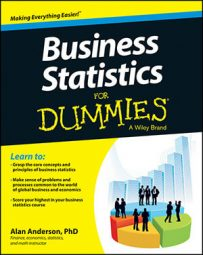The properties of a probability distribution can be summarized with a set of numerical measures known as moments. One of these moments is called the expected value, or mean. In order to calculate an expected value, you use a summation operator.

The summation operator is used to indicate that a set of values should be added together. The formulas used to compute moments for a probability distribution are based on the summation operator. This is because each calculation must be repeated for each possible value of a random variable and the results must be summed.

As an example of the summation operator, suppose a data set contains five elements. The summation operator tells you to perform the following calculations:Xi represents a single element in a data set; i is an index, and n is the number of elements to be summed.

The expected value of a random variable X represents the average value of X that occurs if the random experiment is repeated a large number of times. You can think of the expected value as the center of the distribution.

The expected value is a weighted average of its possible values, with weights equal to probabilities. The formula for computing expected value of X is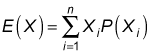Here are the key terms in this formula:

• E(X) = the expected value of X

• n = the number of possible values of X

• i = an index

• Xi = one possible value of X

• P(Xi) = the probability of Xi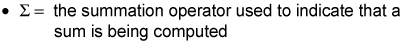Suppose that a biopharmaceutical firm is planning to release several new drugs during the coming year, depending on whether or not the patents are approved. You can use the random variable X to represent the number of new drugs that will be released.

The table shows the probability distribution of these results.

Probability Distribution for Release of New Drugs
X P(X)
0 0.10
1 0.25
2 0.50
3 0.15

You can then use the probability distribution to determine the expected (average) value of X by setting up the possible values of X and the corresponding probabilities, like so:

X1 = 0 P(X1) = 0.10

X2 = 1 P(X2) = 0.25

X3 = 2 P(X3) = 0.50

X4 = 3 P(X4) = 0.15

The corresponding histogram is shown here.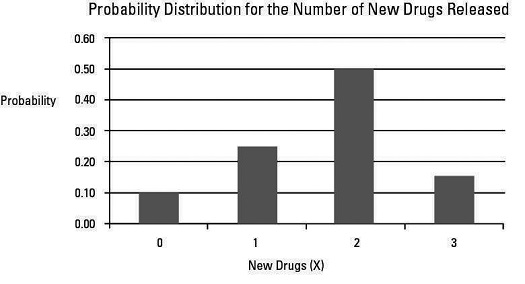Probability distribution for the number of new drugs released.

Next, you substitute these numbers into the expected value formula: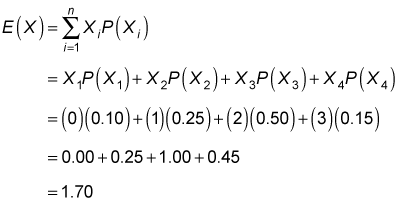This result shows that the expected (average) number of new drugs that will be released during the coming year is 1.7. Although it's physically impossible to release 1.7 new drugs (since 1.7 is not an integer or whole number), if this experiment is repeated many times, the average number of new drugs released will be 1.7.